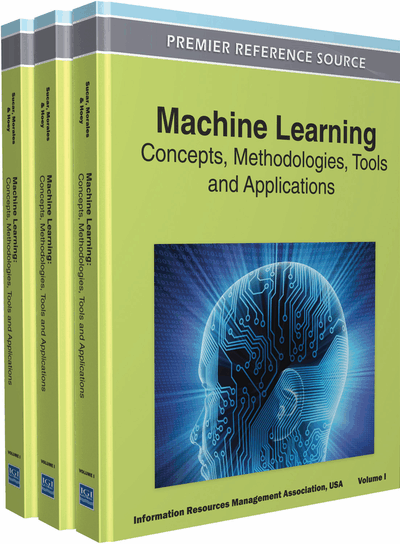# Class Prediction in Test Sets with Shifted Distributions

DOI: 10.4018/978-1-60960-818-7.ch318

## Abstract

Machine learning has provided powerful algorithms that automatically generate predictive models from experience. One specific technique is supervised learning, where the machine is trained to predict a desired output for each input pattern x. This chapter will focus on classification, that is, supervised learning when the output to predict is a class label. For instance predicting whether a patient in a hospital will develop cancer or not. In this example, the class label c is a variable having two possible values, “cancer” or “no cancer”, and the input pattern x is a vector containing patient data (e.g. age, gender, diet, smoking habits, etc.). In order to construct a proper predictive model, supervised learning methods require a set of examples xi together with their respective labels ci. This dataset is called the “training set”. The constructed model is then used to predict the labels of a set of new cases xj called the “test set”. In the cancer prediction example, this is the phase when the model is used to predict cancer in new patients.

One common assumption in supervised learning algorithms is that the statistical structure of the training and test datasets are the same (Hastie, Tibshirani & Friedman, 2001). That is, the test set is assumed to have the same attribute distribution p(x) and same class distribution p(c|x) as the training set. However, this is not usually the case in real applications due to different reasons. For instance, in many problems the training dataset is obtained in a specific manner that differs from the way the test dataset will be generated later. Moreover, the nature of the problem may evolve in time. These phenomena cause pTr(x, c)pTest(x, c), which can degrade the performance of the model constructed in training.

Here we present a new algorithm that allows to re-estimate a model constructed in training using the unlabelled test patterns. We show the convergence properties of the algorithm and illustrate its performance with an artificial problem. Finally we demonstrate its strengths in a heart disease diagnosis problem where the training set is taken from a different hospital than the test set.
Chapter Preview
Top

## Background

In practical problems, the statistical structure of training and test sets can be different, that is, pTr(x, c) ¹ pTest(x, c). This effect can be caused by different reasons. For instance, due to biases in the sampling selection of the training set (Heckman, 1979; Salganicoff, 1997). Other possible cause is that training and test sets can be related to different contexts. For instance, a heart disease diagnosis model that is used in a hospital which is different from the hospital where the training dataset was collected. Then, if the hospitals are located in cities where people have different habits, average age, etc., this will cause a test set with a different statistical structure than the training set.

The special case pTr(x) ¹ pTest(x) and pTr(c | x) = pTest(c | x) is known in the literature as “covariate shift” (Shimodaira, 2000). In the context of machine learning, the covariate shift can degrade the performance of standard machine learning algorithms. Different techniques have been proposed to deal with this problem, see for example (Heckman, 1979; Salganicoff, 1997; Shimodaira, 2000; Sugiyama, Krauledat & Müller, 2007). Transductive learning has also been suggested as another way to improve performance when the statistical structure of the test set is shifted with respect to the training set (Vapnik, 1998; Chen, Wang & Dong, 2003; Wu, Bennett, Cristianini & Shawe-Taylor, 1999).

The statistics of the patterns x can also change in time, for example in a company that has a continuous flow of new and leaving clients (figure 1). If we are interested in constructing a model for prediction, the statistics of the clients when the model is exploited will differ from the statistics in training. Finally, often the concept to be learned is not static but evolves in time (for example, predicting which emails are spam or not), causing pTr(x, c) ¹ pTest(x, c). This problem is known as “concept drift” and different algorithms have been proposed to cope with it (Black & Hickey, 1999; Wang, Fan, Yu, & Han, 2003; Widmer & Kubat, 1996).

Figure 1.

Changes across time of the statistics of clients in a car insurance company. The histograms of two different variables (a, b) related to the clients’ use of their insurance are shown. Dash: data collected four months later than data shown in solid.

## Complete Chapter List

Search this Book:
Reset